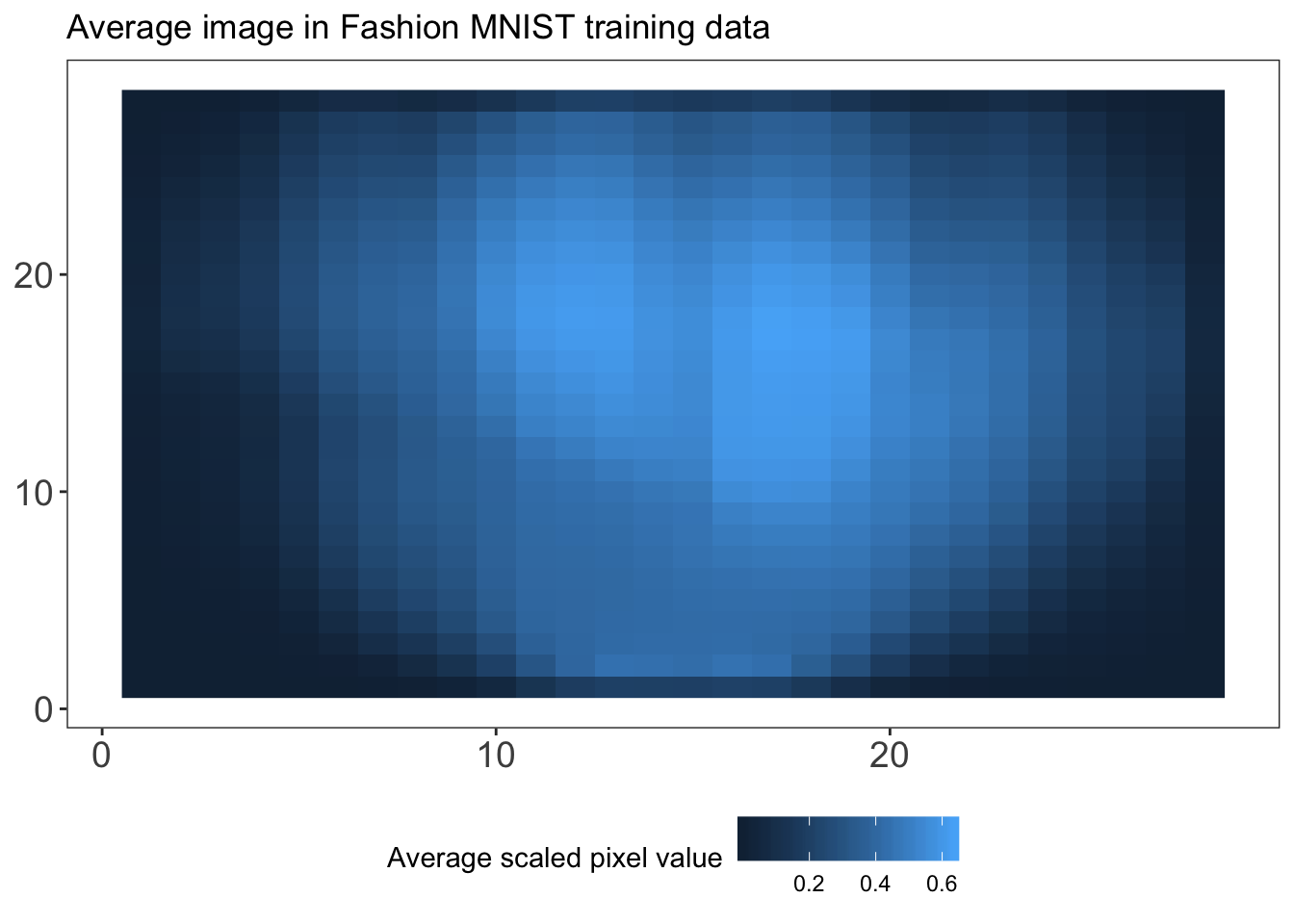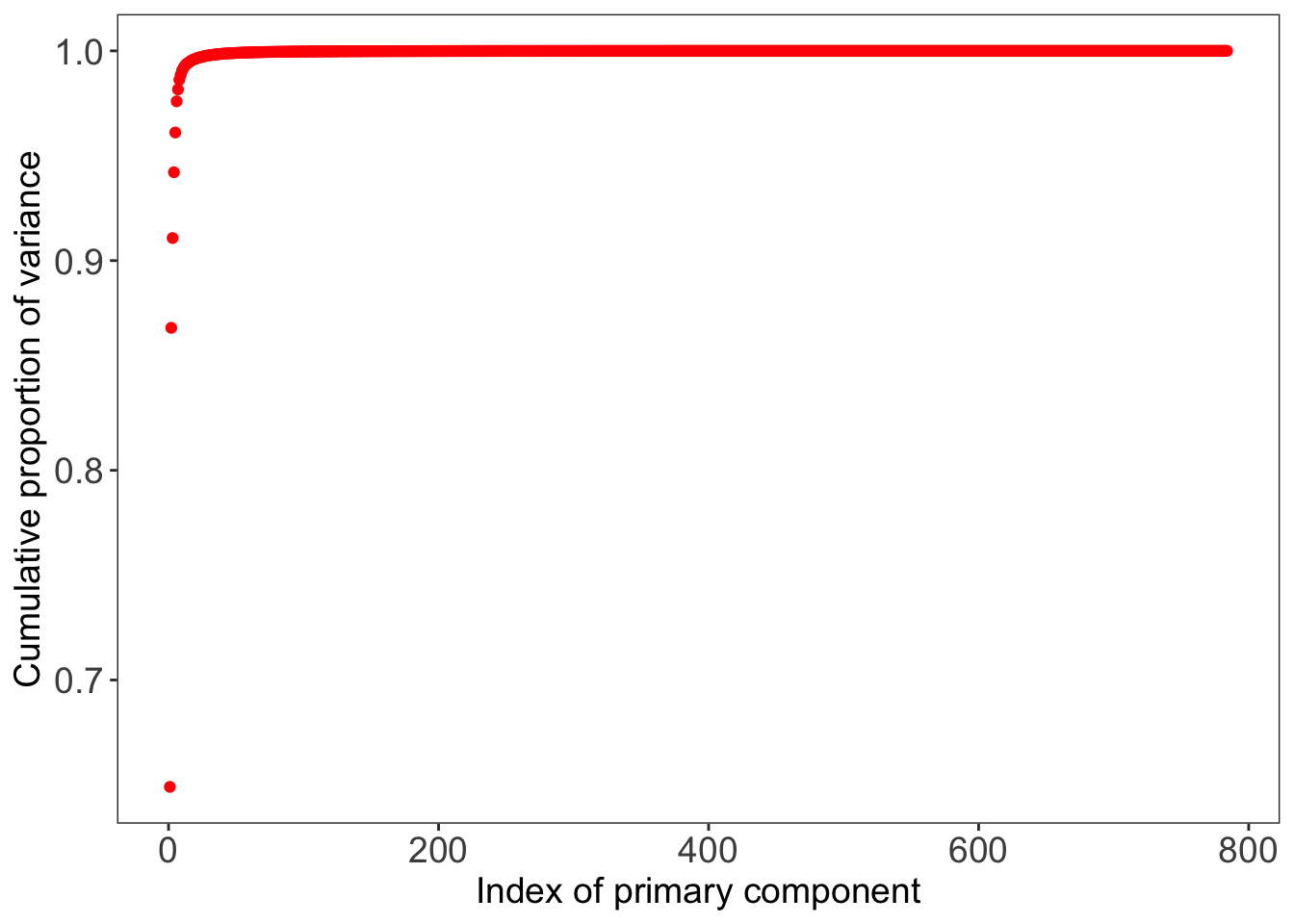# Comparing Machine Learning Algorithms for Predicting Clothing Classes: Part 2

by Florianne Verkroost

Florianne Verkroost is a Ph.D. candidate at Nuffield College at the University of Oxford. She has a passion for data science and a background in mathematics and econometrics. She applies her interdisciplinary knowledge to computationally address societal problems of inequality.

This is the second post in a series devoted to comparing different machine and deep learning methods to predict clothing categories from images using the Fashion MNIST data by Zalando. In the first blog post of this series, we explored the data, prepared the data for analysis and learned how to predict the clothing categories of the Fashion MNIST data using my go-to model: an artificial neural network in Python. In this second blog post, we will perform dimension reduction on the data in order to make it feasible to run standard machine learning models (including tree-based methods and support vector machines) in the future. The R code for the first post can be found on my Github.

library(keras)
library(magrittr)
library(ggplot2)

### Data Preparation

Let’s fetch the data again and prepare the training and test sets.

install_keras()
fashion_mnist = keras::dataset_fashion_mnist()
c(train.images, train.labels) %<-% fashion_mnist$train c(test.images, test.labels) %<-% fashion_mnist$test

Next, we normalize the image data by dividing the pixel values by the maximum value of 255.

train.images = data.frame(t(apply(train.images, 1, c))) / max(fashion_mnist$train$x)
test.images = data.frame(t(apply(test.images, 1, c))) / max(fashion_mnist$train$x)

Now, we combine the training images train.images and labels train.labels as well as test images test.images and labels test.labels in separate data sets, train.data and test.data, respectively.

pixs = ncol(fashion_mnist$train$x)
names(train.images) = names(test.images) = paste0('pixel', 1:(pixs^2))
train.labels = data.frame(label = factor(train.labels))
test.labels = data.frame(label = factor(test.labels))
train.data = cbind(train.labels, train.images)
test.data = cbind(test.labels, test.images)

As train.labels and test.labels contain integer values for the clothing category (i.e. 0, 1, 2, etc.), we also create objects train.classes and test.classes that contain factor labels (i.e. Top, Trouser, Pullover etc.) for the clothing categories. We will need these for some of the machine learning models later on.

cloth_cats = c('Top', 'Trouser', 'Pullover', 'Dress', 'Coat',
'Sandal', 'Shirt', 'Sneaker', 'Bag', 'Boot')
train.classes = factor(cloth_cats[as.numeric(as.character(train.labels$label)) + 1]) test.classes = factor(cloth_cats[as.numeric(as.character(test.labels$label)) + 1])

### Principal Components Analysis

Our training and test image data sets currently contain 784 pixels or variables. We may expect a large share of these pixels, especially those towards the boundaries of the images, to have relatively small variance, because most of the fashion items are centered in the images. In other words, there may be quite some redundant pixels in our data set. To check whether this is the case, let’s plot the average pixel value on a 28 by 28 grid. We first obtain the average pixel values and store these in train.images.ave, after which we plot these values on the grid. We also define a custom plotting theme, my_theme, to make sure all our figures have the same aesthetics. Note that in the plot created, a higher cell (pixel) value means that the average value of that pixel is higher, and thus that the pixel is darker on average (as a pixel value of 0 refers to white and a pixel value of 255 refers to black).

train.images.ave = data.frame(pixel = apply(train.images, 2, mean),
x = rep(1:pixs, each = pixs),
y = rep(1:pixs, pixs))
my_theme = function () {
theme_bw() +
theme(axis.text = element_text(size = 14),
axis.title = element_text(size = 14),
strip.text = element_text(size = 14),
panel.grid.major = element_blank(),
panel.grid.minor = element_blank(),
panel.background = element_blank(),
legend.position = "bottom",
strip.background = element_rect(fill = 'white', colour = 'white'))
}
ggplot() +
geom_raster(data = train.images.ave, aes(x = x, y = y, fill = pixel)) +
my_theme() +
labs(x = NULL, y = NULL, fill = "Average scaled pixel value") +
ggtitle('Average image in Fashion MNIST training data')As we can see from the plot, there are many pixels with a low average value, meaning that they are white in most of the images in our training data. These pixels are mostly redundant, while they do contribute to computational costs and sparsity. Therefore, we might be better off reducing the dimensionality in our data to reduce redundancy, overfitting and computational cost. One method to do so is principal components analysis (PCA). Essentially, PCA statistically reduces the dimensions of a set of correlated variables by transforming them into a smaller number of linearly uncorrelated variables. The resulting “principal components” are linear combinations of the original variables. The first principal component explains the largest part of the variance, followed by the second principal component and so forth. For a more extensive explanation of PCA, I refer you to James et al. (2013).

Let’s have a look at how many variables can explain which part of the variance in our data. We compute the 784 by 784 covariance matrix of our training images using the cov() function, after which we execute PCA on the covariance matrix using the prcomp() function in the stats library. Looking at the results, we observe that 50 principal components in our data explain 99.902% of the variance in the data. This can be nicely shown in a plot of the cumulative proportion of variance against component indices. Note that the component indices here are sorted by their ability to explain the variance in our data, and not based on their pixel position in the 28 by 28 image.

library(stats)
cov.train = cov(train.images)
pca.train = prcomp(cov.train)
plotdf = data.frame(index = 1:(pixs^2),
cumvar = summary(pca.train)$importance["Cumulative Proportion", ]) t(head(plotdf, 50))  ## PC1 PC2 PC3 PC4 PC5 PC6 PC7 PC8 PC9 ## index 1.0000 2.0000 3.0000 4.0000 5.0000 6.0000 7.0000 8.0000 9.0000 ## cumvar 0.6491 0.8679 0.9107 0.9421 0.9611 0.9759 0.9816 0.9862 0.9885 ## PC10 PC11 PC12 PC13 PC14 PC15 PC16 PC17 ## index 10.0000 11.0000 12.0000 13.0000 14.0000 15.0000 16.000 17.0000 ## cumvar 0.9906 0.9918 0.9928 0.9935 0.9941 0.9945 0.995 0.9954 ## PC18 PC19 PC20 PC21 PC22 PC23 PC24 PC25 ## index 18.0000 19.000 20.0000 21.0000 22.0000 23.0000 24.000 25.0000 ## cumvar 0.9957 0.996 0.9962 0.9965 0.9967 0.9969 0.997 0.9972 ## PC26 PC27 PC28 PC29 PC30 PC31 PC32 PC33 ## index 26.0000 27.0000 28.0000 29.0000 30.0000 31.000 32.000 33.0000 ## cumvar 0.9974 0.9975 0.9976 0.9978 0.9979 0.998 0.998 0.9981 ## PC34 PC35 PC36 PC37 PC38 PC39 PC40 PC41 ## index 34.0000 35.0000 36.0000 37.0000 38.0000 39.0000 40.0000 41.0000 ## cumvar 0.9982 0.9983 0.9984 0.9984 0.9985 0.9986 0.9986 0.9987 ## PC42 PC43 PC44 PC45 PC46 PC47 PC48 PC49 ## index 42.0000 43.0000 44.0000 45.0000 46.0000 47.0000 48.000 49.000 ## cumvar 0.9987 0.9988 0.9988 0.9989 0.9989 0.9989 0.999 0.999 ## PC50 ## index 50.000 ## cumvar 0.999 ggplot() + geom_point(data = plotdf, aes(x = index, y = cumvar), color = "red") + labs(x = "Index of primary component", y = "Cumulative proportion of variance") + my_theme() + theme(strip.background = element_rect(fill = 'white', colour = 'black'))We also observe that 99.5% of the variance is explained by only 17 principal components. As 99.5% is already a large share of the variance, and we want to reduce the number of pixels (variables) by as many as we can to reduce computation time for the models coming up, we choose to select these 17 components for further analysis. (Although this is unlikely to influence our results hugely, if you have more time I’d suggest you select the 50 components explaining 99.9% of the data, or execute the analyses on the full data set.) We also save the relevant part of the rotation matrix created by the prcomp() function and stored in pca.train, such that its dimensions become 784 by 17. We then multiply our training and test image data by this rotation matrix called pca.rot. We further combine the transformed image data (train.images.pca and test.images.pca) with the integer labels for the clothing categories in train.data.pca and test.data.pca. We will use these reduced data in our further analyses to decrease computational time. pca.dims = which(plotdf$cumvar >= .995)
pca.rot = pca.train$rotation[, 1:pca.dims] train.images.pca = data.frame(as.matrix(train.images) %*% pca.rot) test.images.pca = data.frame(as.matrix(test.images) %*% pca.rot) train.data.pca = cbind(train.images.pca, label = factor(train.data$label))
test.data.pca = cbind(test.images.pca, label = factor(test.data\$label))

In the next post of this series, we will use the PCA reduced data to estimate and assess tree-based methods, including random forests and gradient-boosted trees.